# Simple Mesh Current Analysis for Electric CircuitsMesh current analysis is used to analyze an electric circuit using the flowing currents in a closed loop of a circuit.

Even we already have Ohm’s law and Kirchhoff’s laws, those two give us more math equations to be solved. Mesh Current Analysis or Maxwell’s Circulating Currents or Loop Current Method is able to lessen the number of equations greatly.

This method is different from the Branch Current method which does not use Kirchhoff’s laws and is supposed to be used for less unknown variables. This mesh analysis is also different with the wye-delta transformation if we don’t have the wye or delta configuration.

Mesh analysis provides us with a different method for analyzing electric circuits using mesh current or loop current as main variables.

Contents

It is more convenient to use mesh current rather than the current flowing through elements in a circuit because we will have fewer equations to be solved.

Take note that a loop is a closed path with no node passed more than once. A mesh is a loop that does not contain any other loop within it.

Now proceed to use these two to develop two powerful techniques for circuit analysis :

1. Nodal analysis
2. Mesh analysis

When nodal analysis uses KCL to determine nodal voltages in a circuit as the main variables, mesh analysis uses KVL to identify mesh current in a circuit.

Keep in mind: mesh current analysis can only be used in the planar circuit. What is planar circuit anyway? It is a circuit that can be redrawn with no branches crossing one another, otherwise, the nonplanar circuit can be seen in Figure.(1).

A circuit may have crossing branches and still be planar if it can be redrawn such that it has no crossing branches like shown in Figure.(2).

## What is Loop and Mesh

We need to completely understand the term of the mesh since we will mention it every time we use this mesh current method. Mesh is basically an ‘exceptional’ loop. I am sure you are well aware of what is a loop in an electrical circuit.

For comparison, take notice of Figure.(3) below:

Let’s refresh that now, what is a loop in an electrical circuit?

Loop is formed by a set of nodes. If the current flows from a starting node and passes through a set of nodes without passing the same node twice, it means the current flows in one loop.

The figure above is clear that the circuit has 3 loops, labelled by clockwise arrows 1, 2, and 3. But, do we also have 3 meshes? That’s the question right there.

Don’t get fooled by the representation of the arrows in the circuit since both loop and mesh are represented by clockwise or counterclockwise arrows.

Mesh generally has the same meaning as a loop, but a mesh is a loop without a loop inside it.

The difference didn’t hit you? Then take note below,

A mesh is a loop which does not contain any other loops within it.

We don’t need further explanation. From Figure.(3) above only loop 1 and loop 2 are considered as meshes because loop 3 is containing other loops (loop 1 and 2). Hence in Figure.(3) we have 3 loops (1, 2, and 3) and 2 meshes (1 and 2).

In Figure.(3) the paths abefa and bcdeb are meshes, but path abcdefa is not a mesh.

Like we read earlier, the current flowing through a mesh or closed-loop is known as mesh current. We will satisfy KVL in every condition when using mesh analysis.

For better and easier understanding, we will forbid the use of the current circuit in mesh analysis this time for a planar circuit. This does not mean mesh analysis is only limited to a voltage source. You will know why down here.

In the mesh analysis of a circuit with n meshes, we use these three following steps.

Steps to Determine Mesh Currents:

1. Assign mesh currents i1, i2, …., in to the n meshes.
2. Apply KVL to each of the n meshes. Use Ohm’s law to express the voltages in terms of the mesh currents.

Solve the resulting n simultaneous equations to get the mesh currents.

## Mesh Current Analysis

Let’s proceed with the mesh current method. We will use the Figure.(3) above. We will assign the voltage sources and resistors with labels as shown below: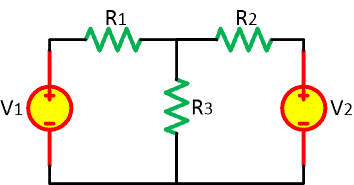And then we apply the meshes with the clockwise direction and then we assign the labels to the currents and polarity for the resistors. Remember that we assign positive polarity to the resistor’s terminal entered by the current for the first time. Because the R3 is in intersect between meshes 1 and 2, we assign i3 to it for now.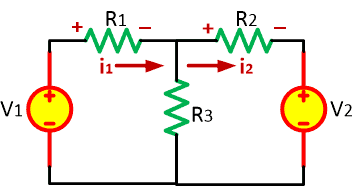We will get the following equations as below:

For mesh 1 (i1):For mesh 2 (i2):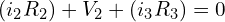And those are the equations for two meshes we have. But how do we solve 3 unknown variables with only 2 equations?

The answer is: THIS WILL BE HARD

But to make things easier, we can write i3 with another equation.

How? Check the mesh current analysis procedure below:

## Mesh Current Analysis Procedure

For you who haven’t learned the mesh current analysis or want to refresh your memory, we will learn it now step-by-step carefully. Not to make things complicated after reading the explanation above, we will still use the Figure.(3) as an example with known variable values.

### Mesh analysis current directions

First, we determine the current directions for each mesh. It is up to you whether they are in clockwise or counterclockwise directions or a mix of them. We will go in a clockwise direction now.### Labelling the circuit elements

We now labelling all the circuit elements with positive and negative polarity depending on the current direction using passive sign convention. For the currents passing the R3, it depends on which mesh we are analyzing at the moment. Below you will find the explanation.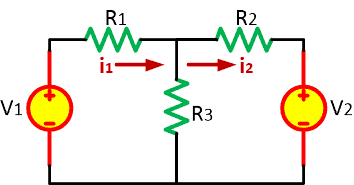### Find the KVL mesh equations

We will write down all the KVL equations to solve the circuit. But before that let’s give the numbers for each variable we have. The final circuit will be:We will use a clockwise direction for each mesh.

For mesh 1,We get:The value of V1 = -3 because the mesh 1 is entering the voltage source terminal from its negative polarity.

For mesh 2,We get:If you are still confused about the currents entering the R3, assume we are analyzing the mesh 1. If we analyze mesh 1, we will prioritize the i1. Look at the figure below.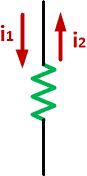For mesh 1, the sum of the currents entering the R3 will be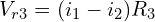If we analyze the mesh 2,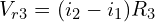Now we have ‘2’ equations for ‘2’ meshes.

For mesh 1,For mesh 2,### Solve the KVL mesh equations

As stated before, for every ‘n’ meshes there will be ‘n’ KVL equations. We have: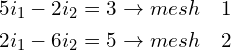We change the mesh 1 equation into: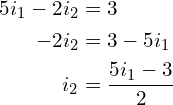Substituting this into the mesh 2 equation,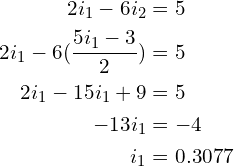Substituting i1 into mesh 1 equation,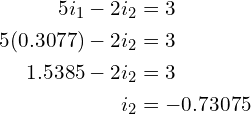It means the direction of i2 is counterclockwise 0.73075 A. With the current values have been discovered, you can find other variables you desire.

## Mesh Current Analysis with Current Source

The example above is the mesh analysis with the voltage source. Now we will analyze a circuit with current sources. It will not be that different from the voltage source, but indeed we need to know the step difference. For example, we will use the circuit below: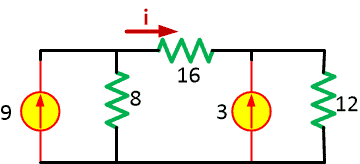We have to find the value of i.

First, we assign the mesh current,We now write down all the KVL equations. We will get 3 equations since there are 3 meshes in the circuit.

For mesh I1,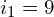Why do we have value without any effort? Because in mesh 1 there is a current source without any intersection with another mesh current.

For mesh I2 and I3,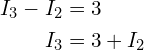When there is a current source in an intersect branch, we call this circuit as a supermesh. The depth of supermesh will be explained in the next post about Supermesh Analysis.

From supermesh:Substituting I3 to this equation results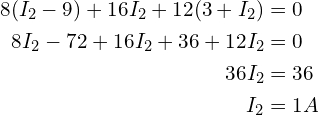Hence,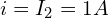## Mesh Analysis 3 Loops

If a circuit has 3 meshes it will be still easy enough to solve as long as there is no current source in an intersection branch with another mesh current. The example below is the mesh analysis 3 loops with an only voltage source and current source in one mesh to make it easier.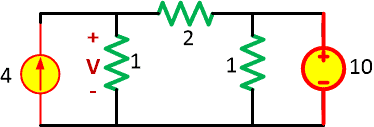We assign the mesh current,For mesh 1,For mesh 2,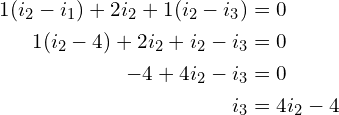For mesh 3,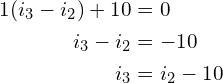Substituting mesh 3 equation into mesh 2,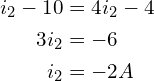Now we have to find the V. The resistor is passed by the mesh current i1 and i2. Since the positive polarity is in top side,## Unbalanced Wheatstone Bridge

Using mesh current analysis is proved easier to use for analyzing an unbalanced Wheatstone bridge. For example, we will use the circuit below: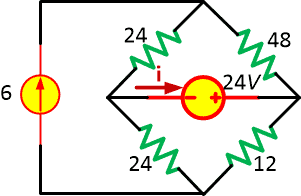We will draw the mesh current inside the loops in a clockwise direction as shown below: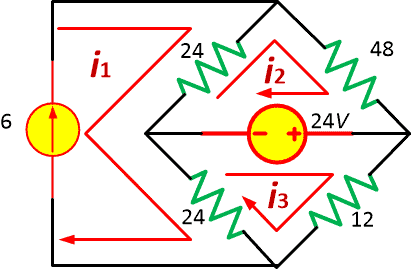As you have seen, we have three meshes and it means we will get three equations. Solving the 2 mesh equations inside the bridge is easier because we have a current source in the mesh 1.

For a start, let’s analyze the current source. From mesh 1,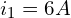From mesh 2,From mesh 3,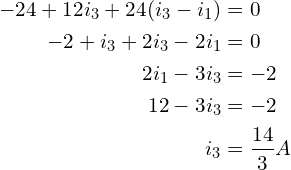Hence,## Mesh Current Analysis Example

It is wise for us to analyze more circuits for better understanding. Let us study the examples below:

1. Find the branch currents value I1, I2, and I3 of the circuit in Figure.(4) below using mesh analysis. Assume the mesh current directions for both loops are in the clockwise direction.

Solution:

First, we write down all the KVL equations. For two meshes we will get two equations:

For mesh 1,

(1.1)For mesh 2,

(1.2)Using substitution,

Substitute (1.2) into (1.1) and we get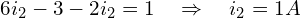From (1.2) we get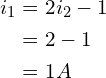Thus,2. Find the value of i in Figure.(5) below using mesh analysis!

Let’s draw the mesh currents in Figure.(6):

For mesh I1,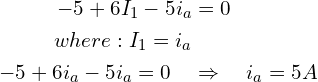For mesh I2,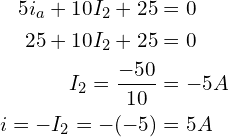### How do you find the current in a mesh analysis?

1. Identify the meshes, (the open windows of the circuit).
2. Assign a current variable to each meshusing a consistent direction (clockwise or counterclockwise).
3. Write Kirchhoff’s Voltage Law equations around each mesh.
4. Solve the resulting system of equations for all mesh currents.

### What are the advantages of using mesh current analysis in solving electronics circuits?

The primary advantage of Mesh Current analysis is that it generally allows for the solution of a large network with fewer unknown values and fewer simultaneous equations. Our example problem took three equations to solve the Branch Current method and only two equations using the Mesh Current method.

### What is the difference between mesh and nodal analysis?

This method differs from the nodal method by using mesh currents instead of nodal voltages as circuit variables. … Nodal method uses Kirchhoff’s currents Law to consider nodal voltages, and Mesh method uses Kirchhoff’s voltages Law to consider mesh currents. Mesh is a loop, which does not contain any other loops.